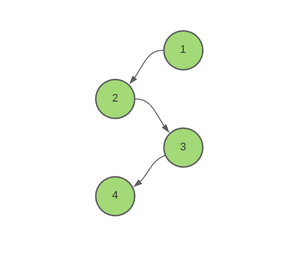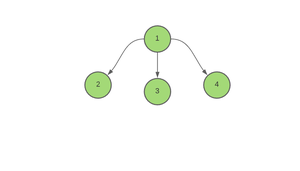Open in App
Not now

# Minimum time required to color all edges of a Tree

• Difficulty Level : Hard
• Last Updated : 22 Oct, 2021

Given an array of pairs Edges[][], representing edges connecting vertices in a Tree consisting of N nodes, the task is to find the minimum time required to color all the edges of a Tree based on the assumption that coloring an edge requires 1 unit of time.

Note: Multiple edges can be colored on a particular instant, but a node can be part of only one of the edges colored on a particular day.

Examples

Input: Edges[][] = ((1, 2), (3, 4), (2, 3))Output: 2
Explanation:
Step 1: Color edges (1, 2) and (3, 4)
Step 2: Color edge (2, 3)

Input: Edges[][] = ((1, 2), (1, 3), (1, 4))Output : 3

Approach: This problem can be solved using DFS(Depth First Search). Follow the steps below to solve the problem:

• Initialize global variables, say ans as 0, to store the minimum time required to color all the edges of a Tree.
• Initialize a variable current_time as 0, to store the time required to color the current edge.
• Iterate over the children of the current node and perform the following steps:
• If the current edge is not visited, i.e the current node is not equal to the parent node:
• Increase current_time by 1.
• Check if the parent edge has been colored at the same time or not. If found to be true, then increase current_time by 1 as a node cannot be part of more than one edge which are being colored at the same time.
• Update ans as maximum of ans and current_time.
• Call the recursive function minTimeToColor for the children of the current node.
• After the end of this function, print ans.

Below is the code for the above approach.

## C++

 `// C++ program for the above approach`   `#include ` `using` `namespace` `std;`   `// Stores the required answer` `int` `ans = 0;`   `// Stores the graph` `vector<``int``> edges;`   `// Function to add edges` `void` `Add_edge(``int` `u, ``int` `v)` `{` `    ``edges[u].push_back(v);` `    ``edges[v].push_back(u);` `}`   `// Function to calculate the minimum time` `// required to color all the edges of a tree` `void` `minTimeToColor(``int` `node, ``int` `parent,` `                    ``int` `arrival_time)` `{` `    ``// Starting from time = 0,` `    ``// for all the child edges` `    ``int` `current_time = 0;`   `    ``for` `(``auto` `x : edges[node]) {`   `        ``// If the edge is not visited yet.` `        ``if` `(x != parent) {`   `            ``// Time of coloring of` `            ``// the current edge` `            ``++current_time;`   `            ``// If the parent edge has` `            ``// been colored at the same time` `            ``if` `(current_time == arrival_time)` `                ``++current_time;`   `            ``// Update the maximum time` `            ``ans = max(ans, current_time);`   `            ``// Recursively call the` `            ``// function to its child node` `            ``minTimeToColor(x, node, current_time);` `        ``}` `    ``}` `}`   `// Driver Code` `int` `main()` `{`   `    ``pair<``int``, ``int``> A[] = { { 1, 2 }, ` `                           ``{ 2, 3 }, ` `                           ``{ 3, 4 } };`   `    ``for` `(``auto` `i : A) {`   `        ``Add_edge(i.first, i.second);` `    ``}`   `    ``// Function call` `    ``minTimeToColor(1, -1, 0);`   `    ``// Finally, print the answer` `    ``cout << ans << ``"\n"``;` `}`

## Java

 `// Java program for the above approach` `import` `java.util.*;`   `class` `GFG{`   `// Stores the required answer` `static` `int` `ans = ``0``;`   `// Stores the graph` `@SuppressWarnings``(``"unchecked"``)` `static` `Vector edges[] = ``new` `Vector[``100000``];`   `// Function to add edges` `static` `void` `Add_edge(``int` `u, ``int` `v)` `{` `    ``edges[u].add(v);` `    ``edges[v].add(u);` `}`   `// Function to calculate the minimum time` `// required to color all the edges of a tree` `static` `void` `minTimeToColor(``int` `node, ``int` `parent,` `                           ``int` `arrival_time)` `{` `    `  `    ``// Starting from time = 0,` `    ``// for all the child edges` `    ``int` `current_time = ``0``;`   `    ``for``(``int` `x = ``0``; x < edges[node].size(); x++) ` `    ``{` `        `  `        ``// If the edge is not visited yet.` `        ``if` `(edges[node].get(x) != parent) ` `        ``{` `            `  `            ``// Time of coloring of` `            ``// the current edge` `            ``++current_time;`   `            ``// If the parent edge has` `            ``// been colored at the same time` `            ``if` `(current_time == arrival_time)` `                ``++current_time;`   `            ``// Update the maximum time` `            ``ans = Math.max(ans, current_time);`   `            ``// Recursively call the` `            ``// function to its child node` `            ``minTimeToColor(edges[node].get(x), node, ` `                           ``current_time);` `        ``}` `    ``}` `}`   `// Driver Code` `public` `static` `void` `main(String[] args)` `{` `    ``for``(``int` `i = ``0``; i < edges.length; i++)` `        ``edges[i] = ``new` `Vector();` `        `  `    ``int` `A[][] = { { ``1``, ``2` `}, ` `                  ``{ ``2``, ``3` `}, ` `                  ``{ ``3``, ``4` `} };`   `    ``for``(``int` `i = ``0``; i < ``3``; i++) ` `    ``{` `        ``Add_edge(A[i][``0``], A[i][``1``]);` `    ``}` `    `  `    ``// Function call` `    ``minTimeToColor(``1``, -``1``, ``0``);`   `    ``// Finally, print the answer` `    ``System.out.print(ans + ``"\n"``);` `}` `}`   `// This code is contributed by umadevi9616`

## Python3

 `# Python3 program for the above approach`   `# Stores the required answer` `ans ``=` `0`   `# Stores the graph` `edges ``=` `[[] ``for` `i ``in` `range``(``100000``)]`   `# Function to add edges` `def` `Add_edge(u, v):` `    `  `    ``global` `edges` `    ``edges[u].append(v)` `    ``edges[v].append(u)`   `# Function to calculate the minimum time` `# required to color all the edges of a tree` `def` `minTimeToColor(node, parent, arrival_time):` `    `  `    ``global` `ans` `    `  `    ``# Starting from time = 0,` `    ``# for all the child edges` `    ``current_time ``=` `0`   `    ``for` `x ``in` `edges[node]:`   `        ``# If the edge is not visited yet.` `        ``if` `(x !``=` `parent):`   `            ``# Time of coloring of` `            ``# the current edge` `            ``current_time ``+``=` `1`   `            ``# If the parent edge has` `            ``# been colored at the same time` `            ``if` `(current_time ``=``=` `arrival_time):` `                ``current_time ``+``=` `1`   `            ``# Update the maximum time` `            ``ans ``=` `max``(ans, current_time)`   `            ``# Recursively call the` `            ``# function to its child node` `            ``minTimeToColor(x, node, current_time)`   `# Driver Code` `if` `__name__ ``=``=` `'__main__'``:` `    `  `    ``A ``=` `[ [ ``1``, ``2` `],` `          ``[ ``2``, ``3` `],` `          ``[ ``3``, ``4` `] ]`   `    ``for` `i ``in` `A:` `        ``Add_edge(i[``0``], i[``1``])`   `    ``# Function call` `    ``minTimeToColor(``1``, ``-``1``, ``0``)`   `    ``# Finally, print the answer` `    ``print``(ans)`   `# This code is contributed by mohit kumar 29`

## C#

 `// C# program for the above approach` `using` `System;` `using` `System.Collections.Generic;` `class` `GFG {` `    `  `    ``// Stores the required answer` `    ``static` `int` `ans = 0;` `     `  `    ``// Stores the graph` `    ``static` `List> edges = ``new` `List>();` `     `  `    ``// Function to add edges` `    ``static` `void` `Add_edge(``int` `u, ``int` `v)` `    ``{` `        ``edges[u].Add(v);` `        ``edges[v].Add(u);` `    ``}` `    `  `    ``// Function to calculate the minimum time` `    ``// required to color all the edges of a tree` `    ``static` `void` `minTimeToColor(``int` `node, ``int` `parent,` `                        ``int` `arrival_time)` `    ``{` `        ``// Starting from time = 0,` `        ``// for all the child edges` `        ``int` `current_time = 0;` `     `  `        ``for``(``int` `x = 0; x < edges[node].Count; x++) {` `     `  `            ``// If the edge is not visited yet.` `            ``if` `(edges[node][x] != parent) {` `     `  `                ``// Time of coloring of` `                ``// the current edge` `                ``++current_time;` `     `  `                ``// If the parent edge has` `                ``// been colored at the same time` `                ``if` `(current_time == arrival_time)` `                    ``++current_time;` `     `  `                ``// Update the maximum time` `                ``ans = Math.Max(ans, current_time);` `     `  `                ``// Recursively call the` `                ``// function to its child node` `                ``minTimeToColor(edges[node][x], node, current_time);` `            ``}` `        ``}` `    ``}`   `  ``// Driver code` `  ``static` `void` `Main() {` `    `  `    ``for``(``int` `i = 0; i < 100000; i++)` `    ``{` `        ``edges.Add(``new` `List<``int``>());` `    ``}` `    `  `    ``int``[,] A = { { 1, 2 }, { 2, 3 }, { 3, 4 } };` ` `  `    ``for``(``int` `i = 0; i < 3; i++) ` `    ``{` `        ``Add_edge(A[i,0], A[i,1]);` `    ``}` ` `  `    ``// Function call` `    ``minTimeToColor(1, -1, 0);` ` `  `    ``// Finally, print the answer` `    ``Console.WriteLine(ans);` `  ``}` `}`   `// This code is contributed by divyeshrabadiya07.`

## Javascript

 ``

Output:

`2`

Time Complexity: O(N)
Auxiliary Space: O(N)

My Personal Notes arrow_drop_up
Related Articles